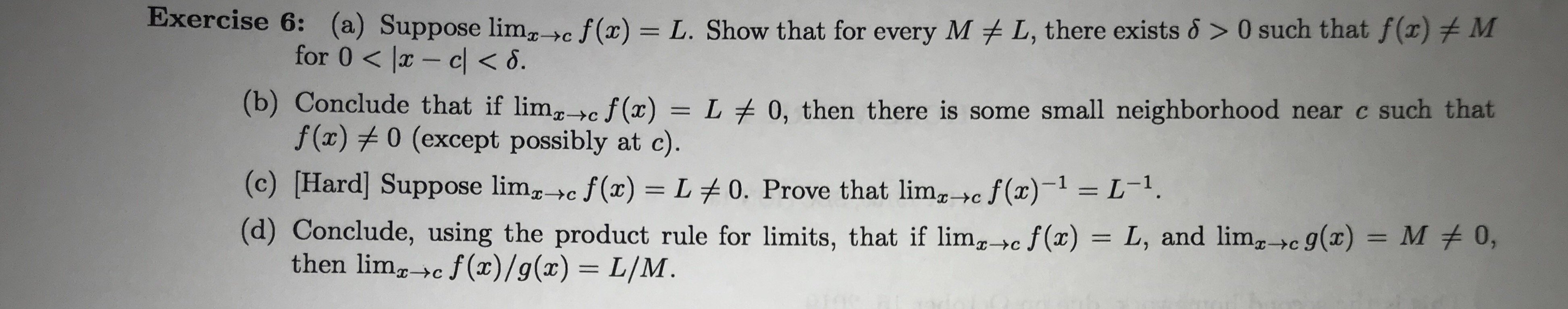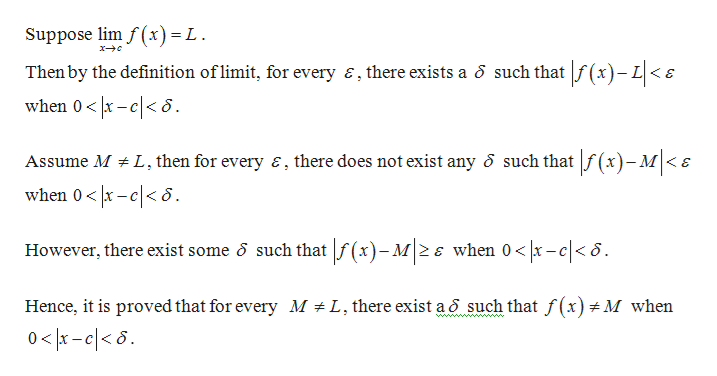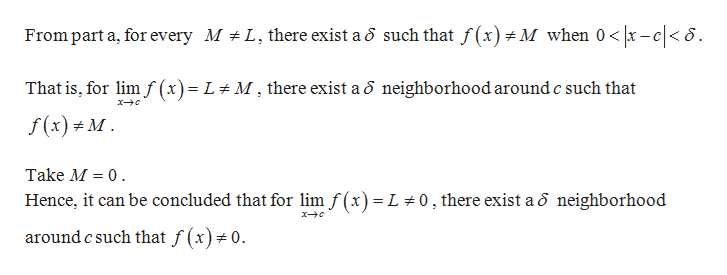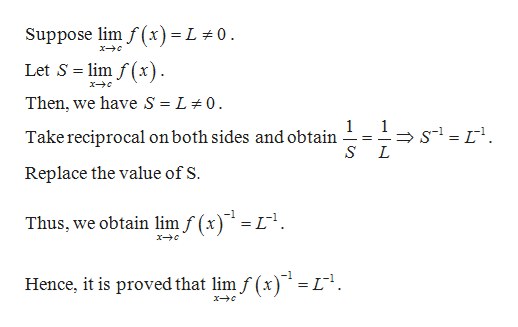# Exercise 6:(a) Suppose lim_>c f(x) = L. Show that for every ML, there exists & > 0 such that f(x)MCfor 0 lx- cl< 6.(b) Conclude that if limcf(x) = L 0, then there is some small neighborhood near c such thatf (x)0 (except possibly at c).(c) [Hard] Suppose limc f(x) L 0. Prove that limc f(x)1 L-.(d) Conclude, using the product rule for limits, that if limg,c f(x) = L, and lim,c g(x) = M # 0,then limgc f(x)/g(x) = L/M.>C

Question
5 viewshelp_outlineImage TranscriptioncloseExercise 6:(a) Suppose lim_>c f(x) = L. Show that for every M L, there exists & > 0 such that f(x) M C for 0 lx- cl< 6. (b) Conclude that if limcf(x) = L 0, then there is some small neighborhood near c such that f (x)0 (except possibly at c). (c) [Hard] Suppose limc f(x) L 0. Prove that limc f(x)1 L-. (d) Conclude, using the product rule for limits, that if limg,c f(x) = L, and lim,c g(x) = M # 0, then limgc f(x)/g(x) = L/M. >C fullscreen
check_circle

star
star
star
star
star
1 Rating
Step 1

a.

Proof:help_outlineImage TranscriptioncloseSuppose lim f (x) = L Then by the definition oflimit, for every E, there exists a 6 such thatf (x)-L
Step 2

b.help_outlineImage TranscriptioncloseFrom part a, for every M L there exist a o such that f(x)M when 0 < x -c<6 That is, for lim f (x) = L M, there exist a o neighborhood around c such that f(x)M Take M 0 Hence, it can be concluded that for lim f (x) = L 0, there exist a 5 neighborhood around c such that f (x)#0. fullscreen
Step 3

c.

Proof:

...help_outlineImage TranscriptioncloseSuppose lim f (x) = L 0 Let S lim f(x) Then, we have S = L 0 1 Take reciprocal on both sides and obtain S L Replace the value of S. Thus, we obtain lim f(x)= L1 x-c -1 Hence, it is proved that limf (x)= L fullscreen

### Want to see the full answer?

See Solution

#### Want to see this answer and more?

Solutions are written by subject experts who are available 24/7. Questions are typically answered within 1 hour.*

See Solution
*Response times may vary by subject and question.
Tagged in

### Math